##### Suplementary notes on select topics in physics and electronics
 Standing waves in a transmission line New Operating modes of 8255A PPI New Select theory of pn junction diode Resonance in series LCR circuit Wave packet and its dynamics Theory of frequency modulation
##### Theory of frequency modulation and phase modulation

In frequency modulation scheme, the frequency of the carrier signal is modulated or changed in accordance with the instanteneous value of the information carrying modulating signal. In phase modulation, on the other hand, it is the phase angle of the carrier signal which is modulated in accordance with the instanteneous value ofthe modulating signal.

###### The carrier signal

We start with a sinusoidally varying signal called the 'carrier', usually of high frequency, and expressed as < br />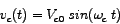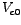is the amplitude and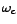is the angular frequency of the carrier signal.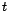is the time.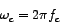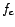is the linear frequency.

###### The modulating signal

Next we consider the message signal containing the information to be transmitted. This signal is called the modulating signal as it is used to modulate or change some charecteristic of the carrier signal. It is of lower frequency vis-a-vis the carrier. The modulating signal may have different forms. We consider here a cosinusoidal modulating signal expressed as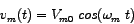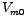is the amplitude and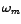is the angular frequency of the modulating signal.is the time.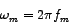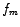is the corresponding linear frequency.

###### The Frequency-Modulated (FM) signal

In order to obtain an expression for the frequency-modulated signal, we start with the instanteneous value of the modulated frequency of the carrier,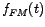, keeping in mind that in frequency modulation the carrier frequency is modulated by the instanteneous value of the modulating signal. By instanteneous, we mean, that which changes with time. The relevant expression would then be: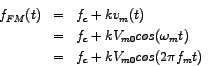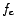is the frequency of the unmodulated carrier,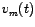is the instanteneous modulating voltage and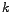is a proportionality constant.

Since the amplitude of the carrier remains fixed in frequency modulation, the form of the instanteneous frequency-modulated signal would be: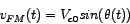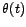is the total instanteneous phase angle of the frequency-modulated voltage waveform. It is correlated withas follows: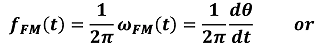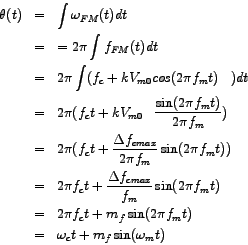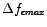is called the maximum frequency deviation in FM and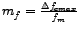is called the modulation index for FM. So finally we arrive at the expression for the frequency-modulated (FM) wave, viz.,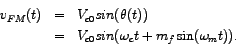###### Side-frequency components of the FM signal

A look at the expression for the frequency-modulated wave, viz.,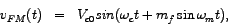which is a sine of a sine function, tells us that it is not easy to determine the frequencies present in the FM wave. However, the frequency-components can be determined, albeit in a complicated way, by expressing the frequency-modulated wave in terms of the so called 'Bessel-functions':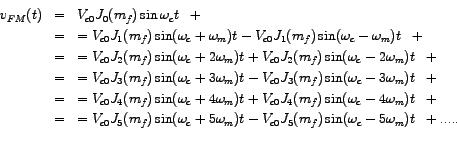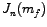are the Bessel-functions of the first kind of order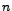(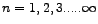).

The values of the Bessel-functions can be obtained from tabulated values and graphs for different values of the modulation-index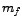. Better still, they can be accurately determined numerically, and this is what has been been done in this work.

From the expression above we observe that the FM wave comprises of a modulated carrier component of frequencyand an infinite number of side-frequency components which may be grouped into, respectively, a pair of 1st-order side-frequency components called 1st-order side-bands, having frequencies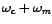and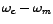, a pair of 2nd-order side-frequency components called 2nd-order sidebands having frequencies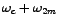and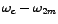, and so on and so forth. The amplitude of each side-frequency component is proportional to the Bessel-function of the corresponding order.

We thus observe that the frequency-components add up to generate the frequency-modulated (FM) waveform. The amplitude of the modulated carrier component is decreased from that of the unmodulated carrier but the decrease is fully compensated by contributions from other side-frequency components. As a result, the amplitude of the FM wave equals that of the unmodulated carrier.

###### The Frequency-Spectrum

The frequency components in a frequency-modulated wave are expressed as vertical lines spaced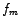apart on both sides of the carrier frequency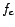in what is called a frequency-spectrum of the FM wave or the FM spectrogram.

The height of each line is proportional to the amplitude of the respective component. The central line depicts the spectral component at the carrier frequency which we call the modulated carrier component. The lines on both sides represent the odd-order and even-order side-frequency component pairs. Each pair of such components is depicted with a line of unique colour in the spectrograms in our right-hand panel. Recall from the expression of the FM wave in terms of its side-frequency components, that the lower frequency component of each odd-order side-frequency pair is always subtracted from the upper frequency component of that pair. On the other hand the lower frequency component of each even-order side-frequency pair is always added from the upper frequency component. This phase relationship between the side-frequency components is expressed in the line spectrum (the first spectrogram in the right-hand panel) by inverting all lines that correspond to the lower frequency componenents of the odd-order frequency component pairs.

However, in a conventional FM spectrogram as shown in the second spectrogram, all the lines are always on the +ve side, since the phase behaviour is considered to be of not much practical importance.
The band-width occupied by the spectrum is given by. Now one observation that comes out very clearly from the spectrgram plots is that the higher-order side-frequency components in general are of reduced amplitude. Taking this into consideration, Carson derived a formula for the band-width by considering only those frequency component pairs that together contribute 96 % of the total radiated power (power is proportional to square of amplitude) which is given below: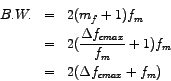It is also interesting to observe how the spectrograms behave when the modulation-indexincreases. Note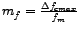where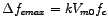. Ifis increased by reducingbut keeping the maximum frequncy deviation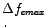constant, then the number of side-frequency components increases without any appreciable increase in bandwidth as is highlighted in the third spectrogram in the right-hand panel. On the other hand, ifis increased by increasingwhile keepingconstant, the band-width does increase appreciably resulting in a sparser line-spectrum.

###### Phase-Modulation (PM)

In phase modulation, as stated at the outset, the instanteneous phase of the carrier is modulated in accordance with the instanteneous value of the modulating signal. The carrier frequency remains constant. We express this mathematically by writing the phase-modulated (PM) carrier as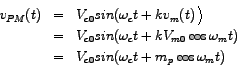Hereis a proportionality constant having dimension of radians per volt. Some use the term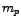in place of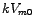and call it the phase-modulation index.
Interestingly, the above expression for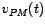can be obtained from the frequency-modulated signal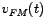by a change of 90 degrees in the phase angle: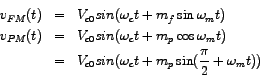is assumed to equal.
We must bear in mind that the analysis above pertains to the simple case of tone-modulation (the modulating signal being a sinusoidal one of single frequency). An analysis for the general case throws up some important conclusions regarding the similarity and difference between FM and PM. A general carrier signal is represented by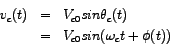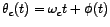is the total instanteneous angle of rotation of the phasor representing the carrier signal voltage.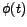is the instanteneous phase (some call it initial phase) of the phasor.
In phase-modulation,changes in accordance with. Thus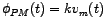,being the modulating or message signal. Thus phase-modulated signal voltage can be expressed as: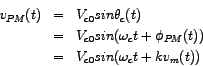Now, the instanteneous frequency of rotation of the carrier-phasor is given by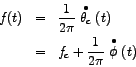For phase modulation (PM), this reduces to: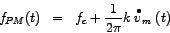For frequency-modulation (FM), on the other hand, we have already seen that the instanteneous frequency is given by: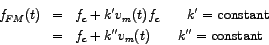Comparing the above equation with the general expression for instanteneous frequency (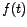) in terms of the instanteneous phase () we have: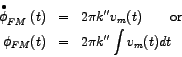This permits us to write the frequency-modulated signal voltage as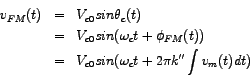So what may we conclude from the above analysis?

1. Frequency and phase modulation are essentially similar to each other.

2. By integrating the modulating or message signal and passing the result through a phase-modulator, we can obtain a frequency-modulated (FM) signal.

3. Conversely, by differentiating the modulating or message signal and passing the result through a frequency-modulator, we can obtain a phase-modulated (PM) signal.

4. FM and PM, both have time-varying i.e. instanteneous phase and frequency. The forms are however different. In fact the nomenclature 'angle-modulation' was coined to mean either of the two.